# A few more questions about fixed point iteration ...?

first of all i simply dont want to give up learning numerical methods ...

i am trying to follow fixed point iteration method from this link ...

fixed point iteration can be used to solve transcendental functions ...

if i am not wrong ,transcendental functions are functions containing trigonometric equations ...

i need a bit of help with trigonometry too .. as i am a bit weak in trigonometry ...

this is a great book to begin maths .. if you are out of touch like myself... i am going to follow this book too ...

Peter Selby, Steve Slavin-Practical Algebra_ A Self-Teaching Guide-John Wiley & Sons (1991)

after that i have plans to move to learn some trigonometry ...

my understanding of trigonometry looks a bit like this only ... unfortunately ...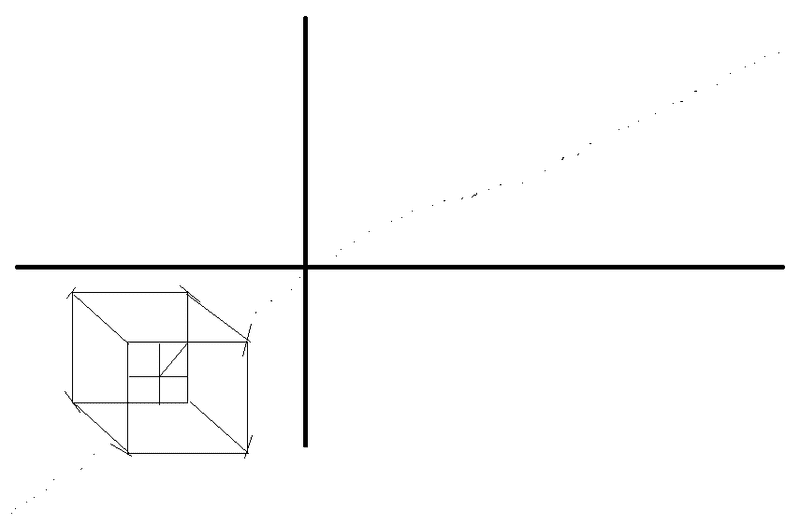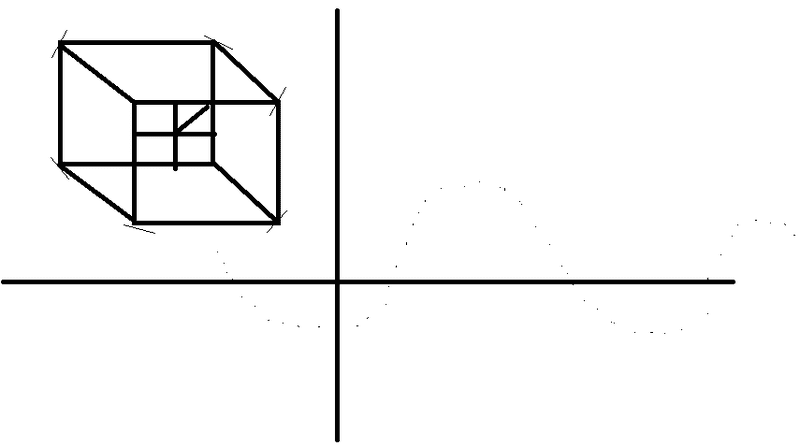solving transcendental functions with the help of numerical methods such as fixed point iteration isn't exactly an easy task for me ...

so i would like to start somewhere ...

can somebody suggest me a good book to learn trigonometry ...???

HallsofIvy
Homework Helper
first of all i simply dont want to give up learning numerical methods ...

i am trying to follow fixed point iteration method from this link ...

fixed point iteration can be used to solve transcendental functions ...

if i am not wrong ,transcendental functions are functions containing trigonometric equations ...
trig functions are transcendental functions but so are exponential functions, logarithms, Bessel functions, etc.

i need a bit of help with trigonometry too .. as i am a bit weak in trigonometry ...

this is a great book to begin maths .. if you are out of touch like myself... i am going to follow this book too ...
What book?

after that i have plans to move to learn some trigonometry ...

my understanding of trigonometry looks a bit like this only ... unfortunately ...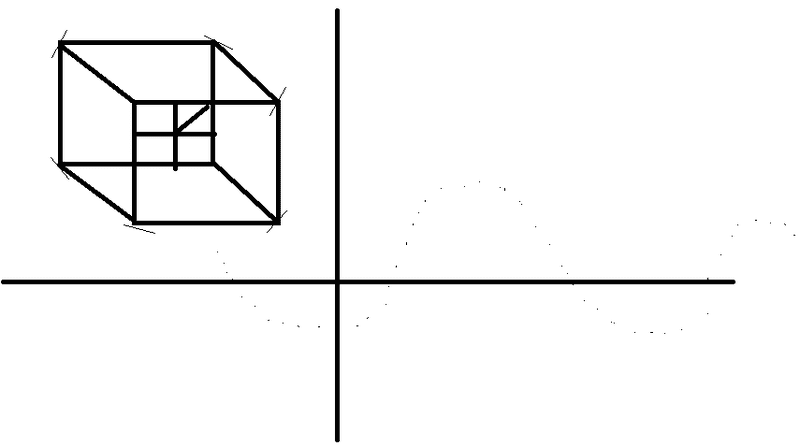I have no idea what those pictures have to do with trigonometry!

solving transcendental functions with the help of numerical methods such as fixed point iteration isn't exactly an easy task for me ...

so i would like to start somewhere ...

can somebody suggest me a good book to learn trigonometry ...???

ok thanks ...

I somehow have to learn this fixed point iteration method properly ...

Geometry and Trigonometry for Calculus: A Self-Teaching Guide
Peter H. Selby

learning it has become the aim of my life ...

I am not going to touch calculus for a very long time too ... I made a path for myself to follow ...

When we know the degree we can also give the polynomial a name:
0 Constant 7
1 Linear 4x+3
3 Cubic 2x^3−5x^2
4 Quartic x^4+3x−2

x+2y-3z=10
2x-3y-4z=1
y-3x+z=-8

in this way I can learn some important numerical methods without touching calculus ...

its like my new aim in life ....

S.G. Janssens
in this way I can learn some important numerical methods without touching calculus ...
In my opinion learning numerical methods without even "touching" calculus is almost impossible, particularly when these methods concern fixed point iteration. Maybe you can learn some numerical linear algebra without calculus, but then I still have my sincere doubts. Actually, my doubts are so serious that I take the liberty to offer you this link: https://www.physicsforums.com/insights/self-study-calculus/

The author of that text offers good advice on learning mathematics, so you might find other texts by him useful, too.
its like my new aim in life ....
I don't know... Learning numerical methods is maybe not the best thing to adopt as your "aim of life". Why don't you aim to be happy and content, alone or together with others, whichever you prefer? Numerical methods will come along the way, if you make a well-planned effort.

actually i was having plans to buy this book too this year for some reference ...

How to Ace Calculus The Streetwise Guide

i found this nice link .. its about numerical methods and it has some examples of fixed point iteration method for different types of equations ...

it was a bit hard to get some sort of flow for this subject .. which is why learning this was a bit depressive ... there are always more pre requisites to fullfill to understand these methods properly ...

http://www.universityofcalicut.info/SDE/BSc_maths_numerical_methods.pdf

from page 11 , of that pdf ... they describe the fixed point iteration method ...

Fixed Point Iteration Method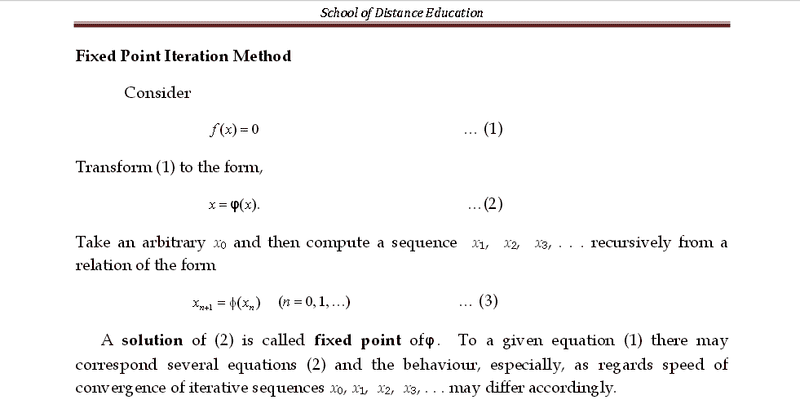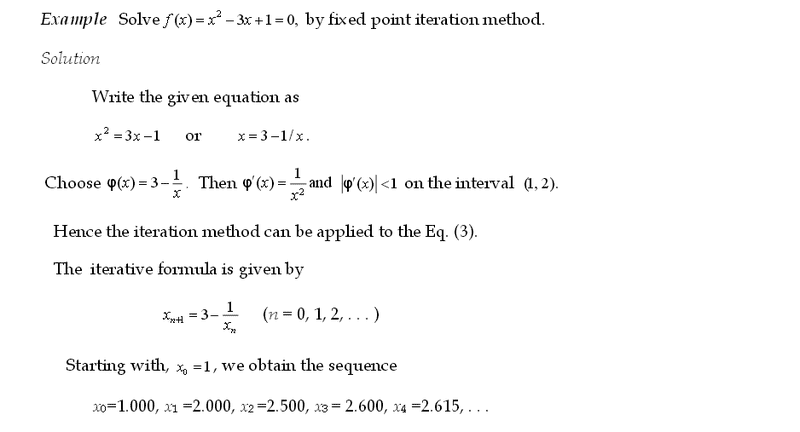i need to understand all those somehow ... it feels better because it has some simple looking equations where this fixed point iteration method can be applied ... even though i dont completly understand the definition .. or why the phi is used ... or what it actually mean by "take an arbitrary x0.....

anyone has time for a rough explanation ???

i managed to narrow it down to few methods ...

linear equations ...

When we know the degree we can also give the polynomial a name:
0 Constant 7
1 Linear 4x+3
3 Cubic 2x^3−5x^2
4 Quartic x^4+3x−2

simultaneous equations ...

x+2y-3z=10
2x-3y-4z=1
y-3x+z=-8

i have a doubt if i am confusing simultaneous equations with systems of linear equations ??
or all these three things .. the linear equation , the systems of linear equations and simultaneous equations different ????

and i think the methods described in these two links below , could be used to solve ... the linear equation , the systems of linear equations and simultaneous equations ....

non linear equations ...

When we know the degree we can also give the polynomial a name:
0 Constant 7
1 Linear 4x+3
3 Cubic 2x^3−5x^2
4 Quartic x^4+3x−2

fixed point iteration
the bisection method
the newton raphson method
the secant method

http://www.maths.dit.ie/~dmackey/lectures/Roots.pdf

these fixed point iteration type methods always involve re arranging equations ... to fit certain formulas ... right ??

do we call the first re arranged form of the equation $$x_n$$
because i dont understand the terms

$$x_0$$
$$x_1$$
$$x_2$$
$$x_3$$
$$x_{n+1}$$

how do i choose this starting value $$x_n$$
is it a common procedure for most of the iteration type numerical methods ??

fixed point iteration
the bisection method
the newton raphson method
the secant method

is it something we get when the equations are re arranged to fit certain formulas ???can somebody help me with the graph of this ones answer .... ??

i am not sure how the graph of the answer looks like ...??

Last edited by a moderator:
NascentOxygen
Staff Emeritus
how do i choose this starting value
You can start with a wild guess, or with a more reasoned guess, or with a rough estimate after trying a few values on your calculator or after sketching a graph.

As a general rule, for a given rearrangement the closer your initial value is to the exact solution, the fewer the required number of iterations to achieve the accuracy desired.

I suggest that you set yourself some exercises using equations for which you already know the exact solution, so you can see how iteration converges towards the solution (or not, as the case may be). For example, you can see in advance the solutions to this quadratic: (x-7)(x+3)=0
which can be written x2 - 4x - 21 = 0

•S.G. Janssens
the things i were following were these numerical methods ...

fixed point iteration
the bisection method
the newton raphson method
the secant method

then i found a simple example to work with ...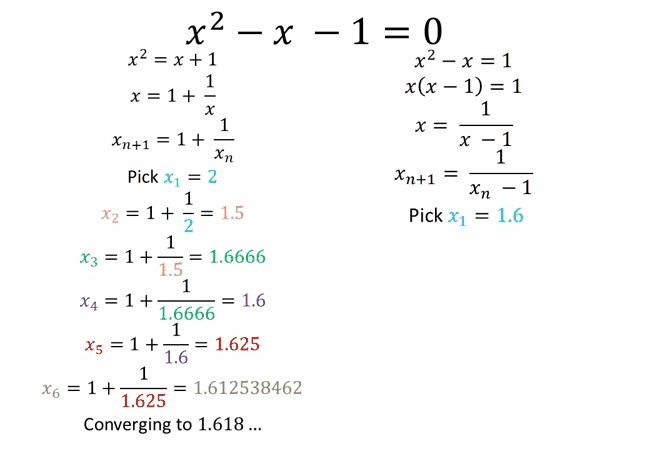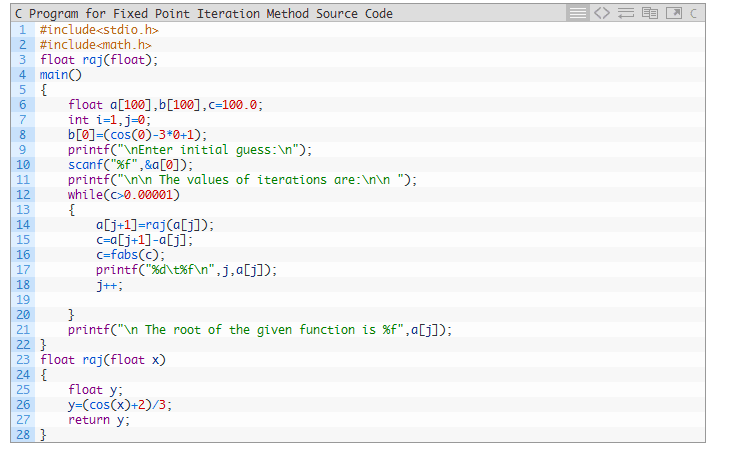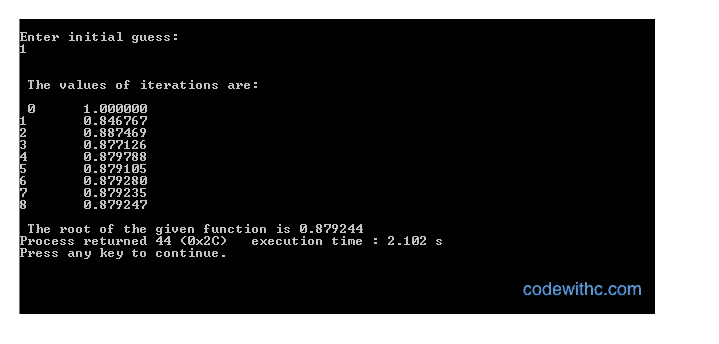then i was trying to figure out a way to solve it ...

i have a little bit of confusions here ...

i think i figured it out that the initial guess ,(the initial root )... the answer of the question ... is like f(1.618) ... which makes the equation close to zero ... ??

this positive root is called a golden ratio ... right ??

should i be looking for more values which makes the equation closer to zero ?

is that why we use numerical methods such as fixed point iteration ... ??

anyway then you put the values to fit the formulas of iteration methods ...

to get more approximate values which makes the equation closer to zero ???

Last edited by a moderator:
NascentOxygen
Staff Emeritus